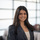# Sorting Stacked Proportional Bar Charts by Magnitude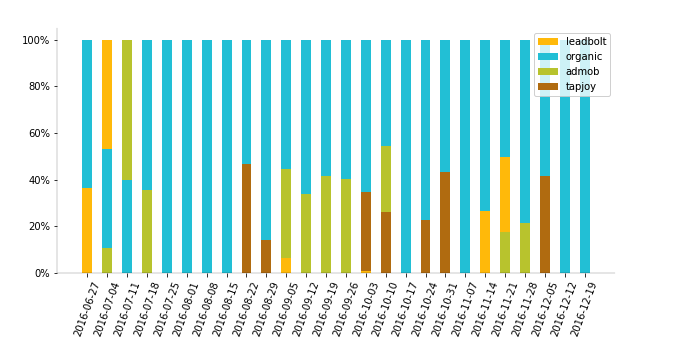By default in many charting libraries and tools, series within stack bars are arranged in the same order. Looking at the image below, blue is above orange, which is above green, which is above purple.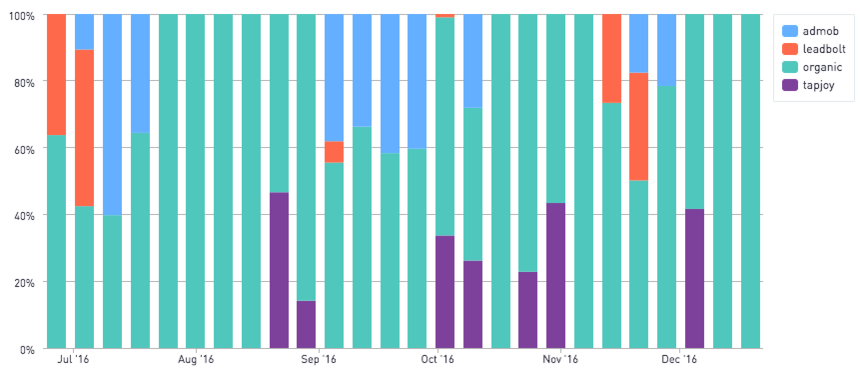But let's say we want these series within each bar to sort by magnitude. In other words, if "organic" was the largest component of a single bar, put organic (green) at the top. If tapjoy is the largest component, show tapjoy (purple) on top.

Matplotib is luckily quite flexible when it comes to charting options. I've worked out a script below using Matplotlib in Periscope's Python/R Integration that generates a stacked proportional bar chart, ordered by magnitude.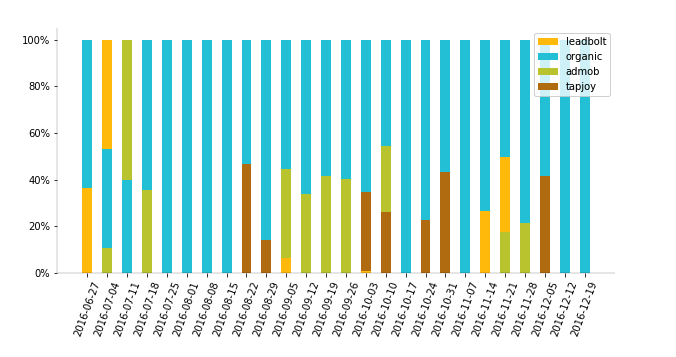Below is my SQL output, containing the week, source of users, and the raw count of users.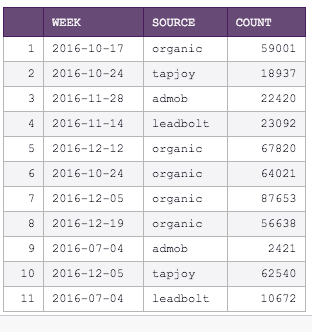Now, I apply the following Python 3.6 script. To repurpose this solution for your own chart, 2 updates are required:

1. Update the helper function to produce the desired color for each of your segments/series
2. in the final line, periscope.output(stacked_bar_by_magnitude(df,'week','count','source')), the first argument of stacked_bar_by_magnitude will be your dataframe, the second argument is the name of the field for your x axis, the third argument is the name of the field for your y axis, and the final argument is the field designating your series.
```# SQL output is imported as a pandas dataframe variable called "df"
import pandas as pd
import numpy as np
import matplotlib.pyplot as plt
from collections import OrderedDict
from matplotlib.ticker import FuncFormatter

###########################################################################################################
### HELPER FUNCTIONS ###
###########################################################################################################

# Function: ranker, ranks entries within a dataframe and adds a colum called rank containing the rank. No updates are required for this function
# Inputs: dataframe to be ranked
# Outputs: ranked data frame. This function is referenced in the Main function below.
def ranker(df1):
"""Equivalent of rownum"""
df1['rank'] = np.arange(len(df1)) + 1
return df1

# Function: color_assigment, assigns colors to each series/segment. Update this function based on your desired coloring scheme and values
# Inputs: dataframe to be assing colors to
# Outputs: colors for each segment. This function is referenced in the Main function below.
def color_assigment(df):
return '#FFC107'
return '#C0CA33'
elif df['source']=='organic':
return '#26C6DA'
else:
return '#B9770E'

###########################################################################################################
### MAIN FUNCTION ###
###########################################################################################################

# Function: stacked_bar_by_magnitude, creates a proportional bar chart where the highest contributing segment is at the top of each bar
# Inputs: (1) dataframe containing the x value, y value and series (2) xaxis - name of the column, in single quotes, of the field to be displayed on the x axis (3) yaxis - name of the column, in single quotes, of the field to be displayed on the y axis (4) series - name of the column, in sigle quotes, which specifies the series/segment that the record corresponds to
# Output: matplotlib image representing a stacked proportional bar chart
#Inspired by https://stackoverflow.com/questions/11273196/stacked-bar-chart-with-differently-ordered-colors-using-matplotlib
def stacked_bar_by_magnitude(df, xaxis, yaxis, series):
df['percentage']=df[yaxis]/df.groupby(xaxis)[yaxis].transform('sum')
df.sort_values('percentage', ascending=False, inplace=True)
df = df.groupby(xaxis).apply(ranker)
df.sort_values([xaxis,'rank'], ascending=[True, False], inplace=True)
df['col']=df.apply(color_assigment,axis=1)
df['bottoms']= df.groupby(xaxis)['percentage'].cumsum() - df['percentage']
df[xaxis]=df[xaxis].astype(str)

fig, ax = plt.subplots()
fig.set_size_inches(10,5)
df = df.reset_index(drop=True)
for i in range(len(df[xaxis])):
ax.bar(df[xaxis][i],df['percentage'][i], width=0.5, color=df['col'][i],bottom=df['bottoms'][i], label=df['source'][i])

plt.xticks(rotation=70)
ax.yaxis.set_major_formatter(FuncFormatter(lambda y, _: '{:.0%}'.format(y)))

ax.spines['left'].set_linewidth(0.2)
ax.spines['bottom'].set_linewidth(0.2)
ax.spines['right'].set_linewidth(0)
ax.spines['top'].set_linewidth(0)

#format legend
handles, labels = plt.gca().get_legend_handles_labels()
by_label = OrderedDict(zip(labels, handles))
plt.legend(by_label.values(), by_label.keys())

return plt

# Use Periscope to visualize a dataframe or an image by passing data to
periscope.output(stacked_bar_by_magnitude(df,'week','count','source'))```